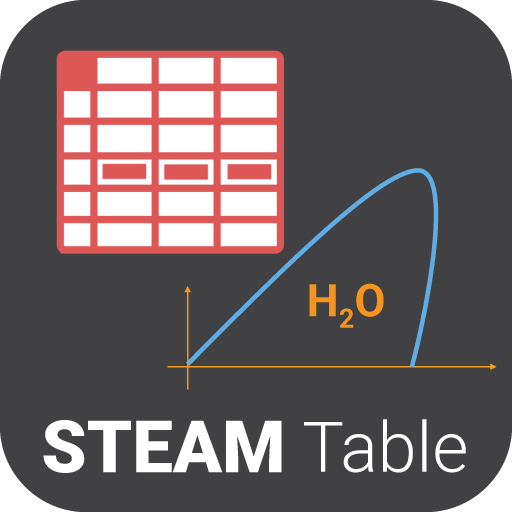Tools Current Version : 1.2 Downloads : 30,723 Size : 3.8 MB

A steam table is an app that helps you calculate water's thermodynamics property using collection of experimental data on temperature, pressure, volume and the energy contained in water and steam. It can be based on a scale of either absolute or relative pressure. Steam Table app helps use to provide the temperature of steam if we know its pressure and inversely, it provide the pressure if we know the temperature. Steam Table also give the volume of water and steam at various temperatures and pressures, which is useful for calculations in thermodynamics.

Steam Table app give us the amount of energy used by water when it changes from liquid to steam and vice versa. Steam Table also provide information about energy changes in steam as it goes from one pressure to another or from one temperature to another.

Some of key calculations here shows:
√ Temperature in Kelvin
√ Pressure in MegaPascal
√ Volume
√ Enthalpy
√ Entropy
√ Isobaric Heat Capacity
√ Isochoric Heat Capacity

Some of the key features of the app are:
? Calculates using Pressure as input. Here you need to enter value of pressure in MPa(a) form. It shows values like Temperature (T) in Kelvin (K), Enthalpy (hg) in kJ/kg, Entropy (sg) in kJ/(kg.K), isobaric heat capacity (Cp) in kJ/(kg.K), and isochoric heat capacity (Cv) in kJ/(kg.K). Here you can select water state i.e. whether it is in vapour or liquid form. It also shows volume (vf) in m^3/kg form.

? Calculates using Temperature as input and calculates Pressure.

? Calculates using Pressure and Temperature as input

? Calculates using Pressure and Vapour Fraction as input

? Calculate using Temperature and Vapour Fraction as input

? App generates Mollier Chart by using the values of enthalpy & entropy calculated using input provided for pressure and/or temperature. Generating h-s diagram for was never so easy before this app.

? Here you can change the units from available options like Engineering, SI, and Imperial. You can also set the number of decimal places from the settings given.

? You can share screenshots of the calculations done with you friends and family members.

Project Members

ASWDC is established by Department of Computer Engineering where students work on live projects under guidance of staff and industry experts. Students are getting extensive knowledge and industrial experience of cutting edge technologies. ASWDC fills gap between academic curriculum and industry expectation.